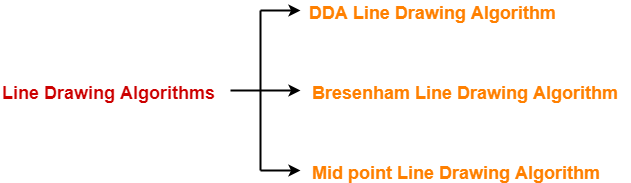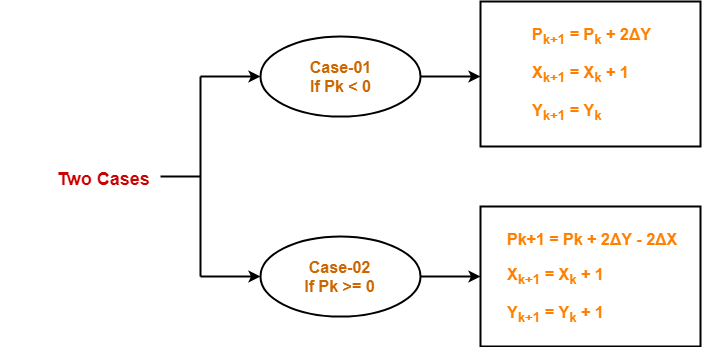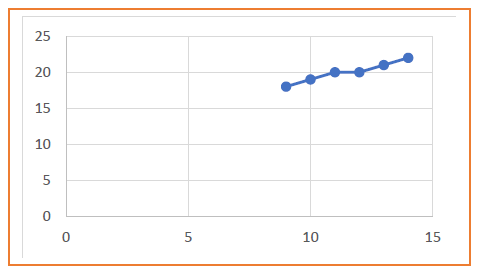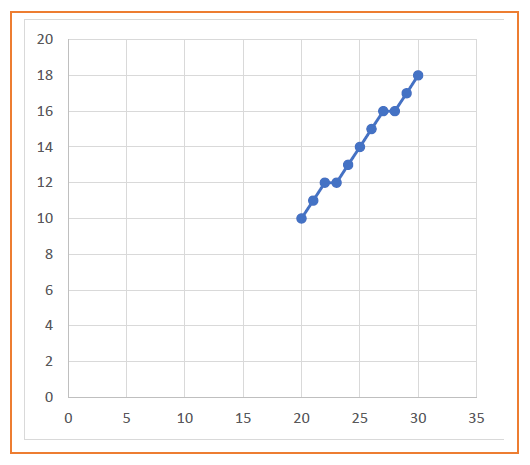# Bresenham Line Drawing Algorithm

## Line Drawing Algorithms-

In computer graphics, popular algorithms used to generate lines are-1. Digital Differential Analyzer (DDA) Line Drawing Algorithm
2. Bresenham Line Drawing Algorithm
3. Mid Point Line Drawing Algorithm

## Bresenham Line Drawing Algorithm-

 Given the starting and ending coordinates of a line, Bresenham Line Drawing Algorithm attempts to generate the points between the starting and ending coordinates.

Also Read- DDA Line Drawing Algorithm

### Procedure-

Given-

• Starting coordinates = (X0, Y0)
• Ending coordinates = (Xn, Yn)

The points generation using Bresenham Line Drawing Algorithm involves the following steps-

### Step-01:

Calculate ΔX and ΔY from the given input.

These parameters are calculated as-

• ΔX = Xn – X0
• ΔY =Yn – Y0

### Step-02:

Calculate the decision parameter Pk.

It is calculated as-

Pk = 2ΔY – ΔX

### Step-03:

Suppose the current point is (Xk, Yk) and the next point is (Xk+1, Yk+1).

Find the next point depending on the value of decision parameter Pk.### Step-04:

Keep repeating Step-03 until the end point is reached or number of iterations equals to (ΔX-1) times.

## Problem-01:

Calculate the points between the starting coordinates (9, 18) and ending coordinates (14, 22).

## Solution-

Given-

• Starting coordinates = (X0, Y0) = (9, 18)
• Ending coordinates = (Xn, Yn) = (14, 22)

### Step-01:

Calculate ΔX and ΔY from the given input.

• ΔX = Xn – X0 = 14 – 9 = 5
• ΔY =Yn – Y0 = 22 – 18 = 4

### Step-02:

Calculate the decision parameter.

Pk

= 2ΔY – ΔX

= 2 x 4 – 5

= 3

So, decision parameter Pk = 3

### Step-03:

As Pk >= 0, so case-02 is satisfied.

Thus,

• Pk+1 = Pk + 2ΔY – 2ΔX = 3 + (2 x 4) – (2 x 5) = 1
• Xk+1 = Xk + 1 = 9 + 1 = 10
• Yk+1 = Yk + 1 = 18 + 1 = 19

Similarly, Step-03 is executed until the end point is reached or number of iterations equals to 4 times.

(Number of iterations = ΔX – 1 = 5 – 1 = 4)

 Pk Pk+1 Xk+1 Yk+1 9 18 3 1 10 19 1 -1 11 20 -1 7 12 20 7 5 13 21 5 3 14 22## Problem-02:

Calculate the points between the starting coordinates (20, 10) and ending coordinates (30, 18).

## Solution-

Given-

• Starting coordinates = (X0, Y0) = (20, 10)
• Ending coordinates = (Xn, Yn) = (30, 18)

### Step-01:

Calculate ΔX and ΔY from the given input.

• ΔX = Xn – X0 = 30 – 20 = 10
• ΔY =Yn – Y0 = 18 – 10 = 8

### Step-02:

Calculate the decision parameter.

Pk

= 2ΔY – ΔX

= 2 x 8 – 10

= 6

So, decision parameter Pk = 6

### Step-03:

As Pk >= 0, so case-02 is satisfied.

Thus,

• Pk+1 = Pk + 2ΔY – 2ΔX = 6 + (2 x 8) – (2 x 10) = 2
• Xk+1 = Xk + 1 = 20 + 1 = 21
• Yk+1 = Yk + 1 = 10 + 1 = 11

Similarly, Step-03 is executed until the end point is reached or number of iterations equals to 9 times.

(Number of iterations = ΔX – 1 = 10 – 1 = 9)

 Pk Pk+1 Xk+1 Yk+1 20 10 6 2 21 11 2 -2 22 12 -2 14 23 12 14 10 24 13 10 6 25 14 6 2 26 15 2 -2 27 16 -2 14 28 16 14 10 29 17 10 6 30 18## Advantages of Bresenham Line Drawing Algorithm-

The advantages of Bresenham Line Drawing Algorithm are-

• It is easy to implement.
• It is fast and incremental.
• It executes fast but less faster than DDA Algorithm.
• The points generated by this algorithm are more accurate than DDA Algorithm.
• It uses fixed points only.

## Disadvantages of Bresenham Line Drawing Algorithm-

The disadvantages of Bresenham Line Drawing Algorithm are-

• Though it improves the accuracy of generated points but still the resulted line is not smooth.
• This algorithm is for the basic line drawing.
• It can not handle diminishing jaggies.

To gain better understanding about Bresenham Line Drawing Algorithm,

Watch this Video Lecture

Next Article- Mid Point Line Drawing Algorithm

Get more notes and other study material of Computer Graphics.

Watch video lectures by visiting our YouTube channel LearnVidFun.

SummaryArticle Name
Bresenham Line Drawing Algorithm
Description
Line Drawing Algorithms- In computer graphics, Bresenham Line Drawing Algorithm is a famous line drawing algorithm. Bresenham Line Drawing Algorithm attempts to generate the points between the starting and ending coordinates.
Author
Publisher Name
Gate Vidyalay
Publisher Logo